Updated On : Dec-05,2019  datascience, datavisulisation, matplotlibMatplotlib - Image Tutorial¶

In :
import matplotlib.pyplot as plt
import matplotlib.image as mpimg
import numpy as np

print(img)
[[[ 1.  1.  1.  0.]
[ 1.  1.  1.  0.]
[ 1.  1.  1.  0.]
...,
[ 1.  1.  1.  0.]
[ 1.  1.  1.  0.]
[ 1.  1.  1.  0.]]

[[ 1.  1.  1.  0.]
[ 1.  1.  1.  0.]
[ 1.  1.  1.  0.]
...,
[ 1.  1.  1.  0.]
[ 1.  1.  1.  0.]
[ 1.  1.  1.  0.]]

[[ 1.  1.  1.  0.]
[ 1.  1.  1.  0.]
[ 1.  1.  1.  0.]
...,
[ 1.  1.  1.  0.]
[ 1.  1.  1.  0.]
[ 1.  1.  1.  0.]]

...,
[[ 1.  1.  1.  0.]
[ 1.  1.  1.  0.]
[ 1.  1.  1.  0.]
...,
[ 1.  1.  1.  0.]
[ 1.  1.  1.  0.]
[ 1.  1.  1.  0.]]

[[ 1.  1.  1.  0.]
[ 1.  1.  1.  0.]
[ 1.  1.  1.  0.]
...,
[ 1.  1.  1.  0.]
[ 1.  1.  1.  0.]
[ 1.  1.  1.  0.]]

[[ 1.  1.  1.  0.]
[ 1.  1.  1.  0.]
[ 1.  1.  1.  0.]
...,
[ 1.  1.  1.  0.]
[ 1.  1.  1.  0.]
[ 1.  1.  1.  0.]]]
In :
import matplotlib.pyplot as plt
import matplotlib.image as mpimg
import numpy as np

imgplot = plt.imshow(img)
plt.show()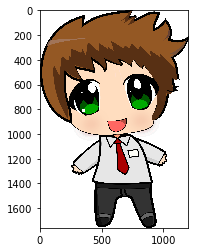In :
import matplotlib.pyplot as plt
import matplotlib.image as mpimg
import numpy as np

lum_img = img[:, :, 0]
plt.imshow(lum_img)

plt.show()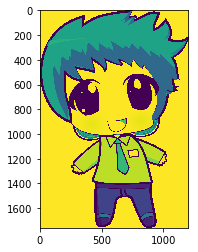In :
import matplotlib.pyplot as plt
import matplotlib.image as mpimg
import numpy as np

lum_img = img[:, :, 0]
plt.imshow(lum_img, cmap="hot")

plt.show()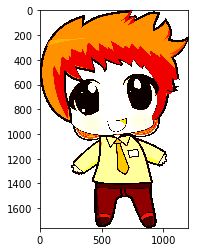In :
import matplotlib.pyplot as plt
import matplotlib.image as mpimg
import numpy as np

lum_img = img[:, :, 0]
plt.imshow(lum_img, cmap="nipy_spectral")

plt.show()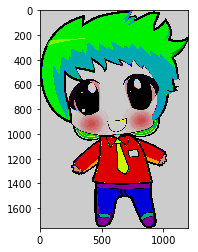In :
import matplotlib.pyplot as plt
import matplotlib.image as mpimg
import numpy as np

lum_img = img[:, :, 0]
plt.imshow(lum_img, cmap="nipy_spectral")
plt.colorbar()

plt.show()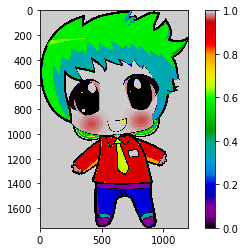Examining A Specific Data Range¶

Sometimes you want to enhance the contrast in your image or expand the contrast in a particular region while sacrificing the detail in colors that don't vary much or don't matter. A good tool to find interesting regions is the histogram. To create a histogram of our image data, we use the hist() function.

In :
import matplotlib.pyplot as plt
import matplotlib.image as mpimg
import numpy as np

lum_img = img[:, :, 0]
plt.imshow(lum_img)
plt.hist(lum_img.ravel(), bins=256, range=(0.0, 5.0))
plt.show()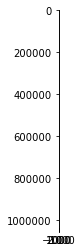In :
import matplotlib.pyplot as plt
import matplotlib.image as mpimg
import numpy as np

fig = plt.figure()
imgplot = plt.imshow(lum_img)
a.set_title('Before')
plt.colorbar(ticks=[0.1, 0.3, 0.5, 0.7], orientation='horizontal')
imgplot = plt.imshow(lum_img)
imgplot.set_clim(0.0, 0.7)
a.set_title('After')
plt.colorbar(ticks=[0.1, 0.3, 0.5, 0.7], orientation='horizontal')

plt.show()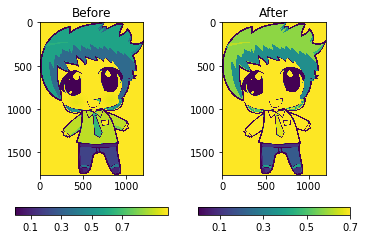Array Interpolation Schemes¶

Interpolation calculates what the color or value of a pixel "should" be, according to different mathematical schemes. One commonplace that this happens is when you resize an image. The number of pixels change, but you want the same information. Since pixels are discrete, there's missing space. Interpolation is how you fill that space. This is why your images sometimes come out looking pixelated when you blow them up. The effect is more pronounced when the difference between the original image and the expanded image is greater. Let's take our image and shrink it. We're effectively discarding pixels, only keeping a select few. Now when we plot it, that data gets blown up to the size on your screen. The old pixels aren't there anymore, and the computer has to draw in pixels to fill that space.

We'll use the Pillow library that we used to load the image also to resize the image.

In :
from PIL import Image

img = Image.open('/home/dolly/Desktop/tutorials/test2.png')
img.thumbnail((64, 64), Image.ANTIALIAS)  # resizes image in-place
imgplot = plt.imshow(img)
plt.show()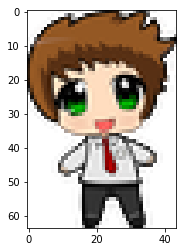In :
from PIL import Image

img = Image.open('/home/dolly/Desktop/tutorials/test2.png')
img.thumbnail((64, 64), Image.ANTIALIAS)  # resizes image in-place
imgplot = plt.imshow(img, interpolation="nearest")
plt.show()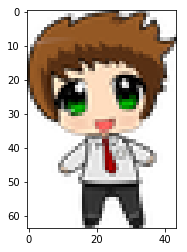In :
from PIL import Image

img = Image.open('/home/dolly/Desktop/tutorials/test2.png')
img.thumbnail((100, 100), Image.ANTIALIAS)  # resizes image in-place
imgplot = plt.imshow(img, interpolation="nearest")
plt.show()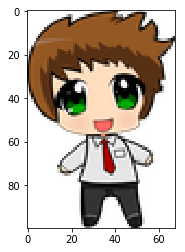In :
from PIL import Image

img = Image.open('/home/dolly/Desktop/tutorials/test2.png')
img.thumbnail((100, 100), Image.ANTIALIAS)  # resizes image in-place
imgplot = plt.imshow(img, interpolation="bicubic")
plt.show()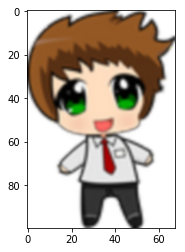Working With Watermark Image¶

In :
import numpy as np

import matplotlib.image as image
import matplotlib.pyplot as plt

# Fixing random state for reproducibility
np.random.seed(19680801)

datafile[:, :, -1] = 0.5  # set the alpha channel

fig, ax = plt.subplots()

ax.plot(np.random.rand(20), '-o', ms=20, lw=2, alpha=0.7, mfc='orange')
ax.grid()
fig.figimage(im, 10, 10, zorder=3)

plt.show()
[ 1.  1.  1.  0.]
[ 1.  1.  1.  0.]
...,
[ 1.  1.  1.  0.]
[ 1.  1.  1.  0.]
[ 1.  1.  1.  0.]]

[[ 1.  1.  1.  0.]
[ 1.  1.  1.  0.]
[ 1.  1.  1.  0.]
...,
[ 1.  1.  1.  0.]
[ 1.  1.  1.  0.]
[ 1.  1.  1.  0.]]

[[ 1.  1.  1.  0.]
[ 1.  1.  1.  0.]
[ 1.  1.  1.  0.]
...,
[ 1.  1.  1.  0.]
[ 1.  1.  1.  0.]
[ 1.  1.  1.  0.]]

...,
[[ 1.  1.  1.  0.]
[ 1.  1.  1.  0.]
[ 1.  1.  1.  0.]
...,
[ 1.  1.  1.  0.]
[ 1.  1.  1.  0.]
[ 1.  1.  1.  0.]]

[[ 1.  1.  1.  0.]
[ 1.  1.  1.  0.]
[ 1.  1.  1.  0.]
...,
[ 1.  1.  1.  0.]
[ 1.  1.  1.  0.]
[ 1.  1.  1.  0.]]

[[ 1.  1.  1.  0.]
[ 1.  1.  1.  0.]
[ 1.  1.  1.  0.]
...,
[ 1.  1.  1.  0.]
[ 1.  1.  1.  0.]
[ 1.  1.  1.  0.]]]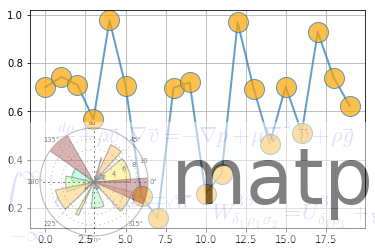Clipping Images With Patches¶

In :
import matplotlib.pyplot as plt
import matplotlib.patches as patches

fig, ax = plt.subplots()
im = ax.imshow(img)
patch = patches.Circle((360, 300), radius=800, transform=ax.transData)
im.set_clip_path(patch)

ax.axis('off')
plt.show()In :
import matplotlib.pyplot as plt
import numpy as np

from matplotlib.patches import Circle
from matplotlib.offsetbox import (TextArea, DrawingArea, OffsetImage,
AnnotationBbox)
from matplotlib.cbook import get_sample_data

if 1:
fig, ax = plt.subplots()

# Define a 1st position to annotate (display it with a marker)
xy = (0.5, 0.7)
ax.plot(xy, xy, ".r")

# Annotate the 1st position with a text box ('Test 1')
offsetbox = TextArea("Test 1", minimumdescent=False)

ab = AnnotationBbox(offsetbox, xy,
xybox=(-20, 40),
xycoords='data',
boxcoords="offset points",
arrowprops=dict(arrowstyle="->"))

# Annotate the 1st position with another text box ('Test')
offsetbox = TextArea("Test", minimumdescent=False)

ab = AnnotationBbox(offsetbox, xy,
xybox=(1.02, xy),
xycoords='data',
boxcoords=("axes fraction", "data"),
box_alignment=(0., 0.5),
arrowprops=dict(arrowstyle="->"))

# Define a 2nd position to annotate (don't display with a marker this time)
xy = [0.3, 0.55]

# Annotate the 2nd position with a circle patch
da = DrawingArea(20, 20, 0, 0)
p = Circle((10, 10), 10)

ab = AnnotationBbox(da, xy,
xybox=(1.02, xy),
xycoords='data',
boxcoords=("axes fraction", "data"),
box_alignment=(0., 0.5),
arrowprops=dict(arrowstyle="->"))

# Annotate the 2nd position with an image (a generated array of pixels)
arr = np.arange(100).reshape((10, 10))
im = OffsetImage(arr, zoom=2)
im.image.axes = ax

ab = AnnotationBbox(im, xy,
xybox=(-50., 50.),
xycoords='data',
boxcoords="offset points",
arrowprops=dict(arrowstyle="->"))

# Annotate the 2nd position with another image (a Grace Hopper portrait)
fn = get_sample_data("grace_hopper.png", asfileobj=False)

imagebox = OffsetImage(arr_img, zoom=0.2)
imagebox.image.axes = ax

ab = AnnotationBbox(imagebox, xy,
xybox=(120., -80.),
xycoords='data',
boxcoords="offset points",
arrowprops=dict(
arrowstyle="->",
)

# Fix the display limits to see everything
ax.set_xlim(0, 1)
ax.set_ylim(0, 1)

plt.show()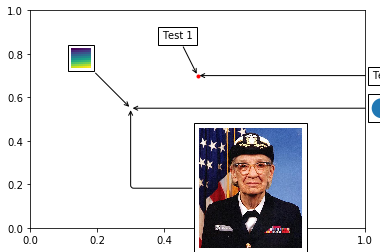In :
import numpy as np
import matplotlib.pyplot as plt
import matplotlib as mpl
plt.style.use('ggplot')
data = np.random.randn(50)
print(plt.style.available)
[u'seaborn-darkgrid', u'seaborn-notebook', u'classic', u'seaborn-ticks', u'grayscale', u'bmh', u'seaborn-talk', u'dark_background', u'ggplot', u'fivethirtyeight', u'_classic_test', u'seaborn-colorblind', u'seaborn-deep', u'seaborn-whitegrid', u'seaborn', u'seaborn-poster', u'seaborn-bright', u'seaborn-muted', u'seaborn-paper', u'seaborn-white', u'seaborn-pastel', u'seaborn-dark', u'seaborn-dark-palette']
In :
import matplotlib.pyplot as plt
import numpy as np
#plt.style.use(['dark_background', 'presentation'])
with plt.style.context(('dark_background')):
plt.plot(np.sin(np.linspace(0, 2 * np.pi)), 'r-o')
plt.show()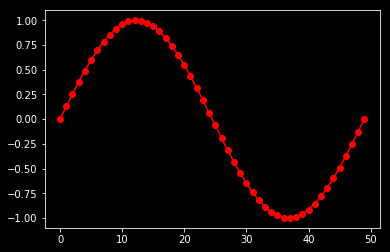Support Us to Make a Difference

Thank You for visiting our website. If you like our work, please support us so that we can keep on creating new tutorials/blogs on interesting topics (like AI, ML, Data Science, Python, Digital Marketing, SEO, etc.) that can help people learn new things faster. You can support us by clicking on the Coffee button at the bottom right corner. We would appreciate even if you can give a thumbs-up to our article in the comments section below.

Want to Share Your Views? Have Any Suggestions?

If you want to

• provide some suggestions on topic